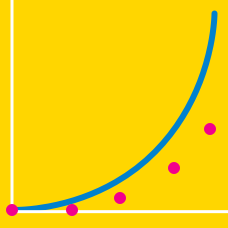Quantitative Finance

Newton Raphson Method

Let $f(x) = x^3 - 72x - 220.$ Given that $f(x)=0$ has a root near $x = 12,$ compute $x_1$ by using Newton-Raphson method starting with $x_0=12.$

By using the Newton-Raphson method, find the positive root of the following quadratic equation correct to $5$ significant figures: $x^2 + 9x - 5 = 0.$

Start with $x_0 = 2.2.$

Use the Newton-Raphson method, correct to $5$ significant figures, to get the root near $x = 2$ of the equation $f(x) = x^3 - 3x - 8.$

The equation $f(x) = 4e^x - 12$ has a root near $x = 1.$ Use the Newton-Raphson method to calculate the root correct to $5$ significant figures.

Let $f(x) = 4 x^2 - 8.$ Which of the following is the correct representation of $x_{n+1}$ that Newton-Raphson method leads to?

×